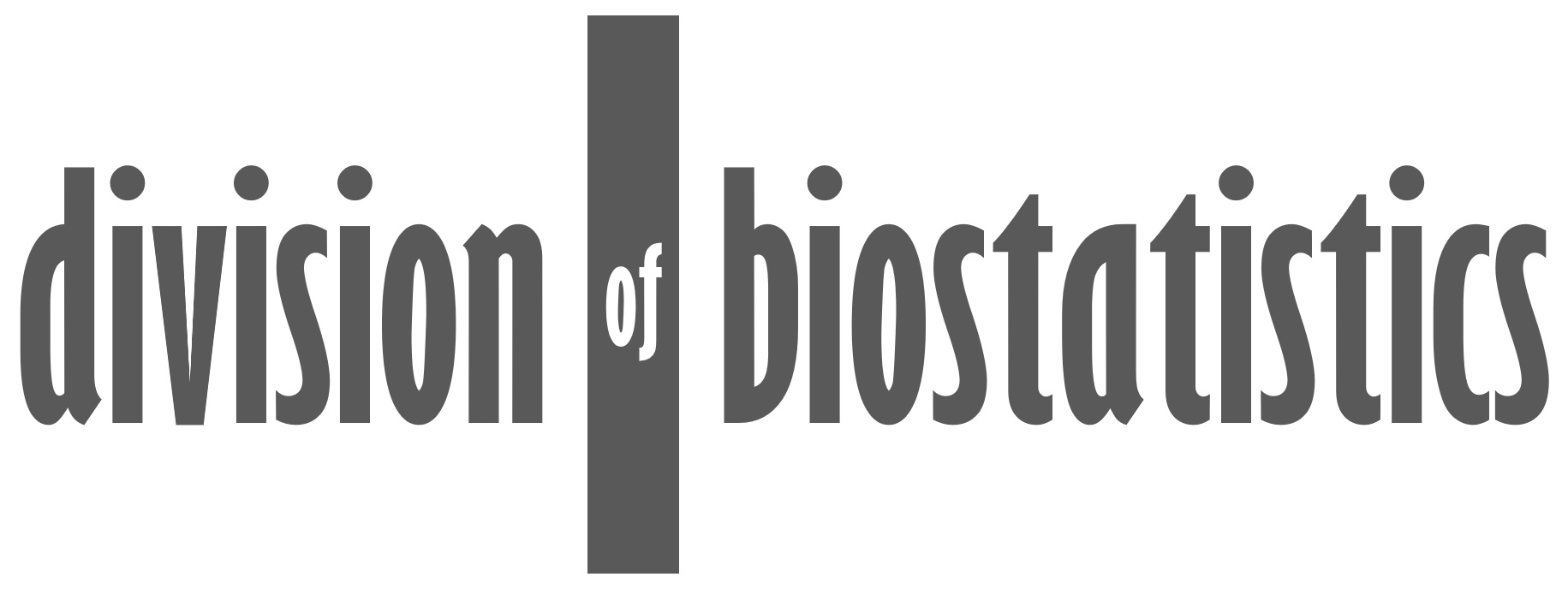Parametric Quantile Models Quantile regression models conditional quantiles of outcome variables of interest given covariates. Its regression coefficients depend on the order of the quantile being estimated. For example, the coefficients for the median are generally different from those of the 10th centile. We have developed parametric quantile models. These are statistically efficient and may expand the potential of statistical modeling. Goodness-of-fit measures and testing procedures are available. Papers Frumento P, Bottai M. Parametric modeling of quantile regression coefficient functions. Biometrics. 72: 74-84, 2016. Frumento P, Bottai M. Parametric modeling of quantile regression coefficient functions with censored and truncated data. Biometrics. 73: 1179-1188, 2017 Bottai M, Orsini N. qmodel: a command for fitting parametric quantile models. The Stata Journal, 19(2): 261-293, 2019 Sottile G, Frumento P, Chiodi M, Bottai M. A penalized approach to covariate selection through quantile regression coefficient models. Statistical Modelling. 2019 Bottai M, Cilluffo G. Nonlinear parametric quantile models. Statistical Methods in Medical Research. 2020 Frumento P, Bottai M, Fernández-Val I. Parametric modeling of quantile regression coefficient functions with longitudinal data. Journal of the American Statistical Association, 2021 (to appear) Software R package: "qrcm" R package: "ctqr" Download with the following Stata commands: net from http://www.imm.ki.se/biostatistics/stata net install qmodel, replace Presentations September 1, 2017 The new qcm command for nonlinear quantile coefficient models 2017 Nordic and Baltic Stata Users Group Meeting, Stockholm, Sweden July 15, 2016 Parametric modeling of quantile regression coefficient functions with censored and truncated data Workshop on Recent Advances in Quantile and M-quantile Regression, Pisa, Italy January 8, 2015 Parametric modeling of quantile regression coefficients Dep. Mathematics, University of Cagliari, Italy November 12, 2014 Parametric modeling of quantile regression coefficient functions Gothenburg University, Gothenburg, Sweden